# Two dimensional Gaussian blur of slanted edge

As a model of line spread of slanted edge consider the function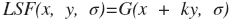where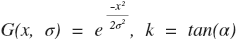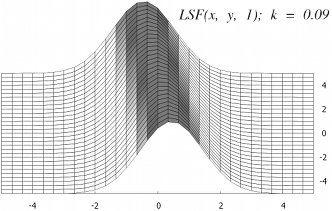Fig 1a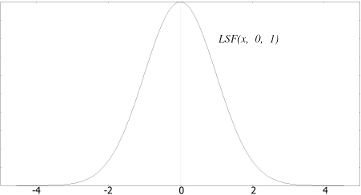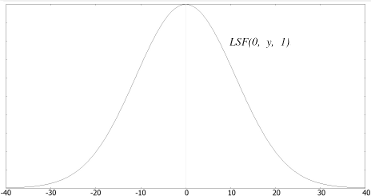Fig 1b Fig 1c

Fig 1a - LSF with sigma = 1 and k = 0.09; Fig 1b - xz section at y = 0; Fig 1c - yz section at x = 0;

Consider applying 2d Gaussian blur with sigma =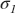to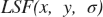. Regarding the Gaussian blur's separability we can represent it as two sequenced convolutions in x and y directions: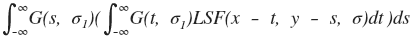Eq. 1

Gaussian blur has two more properties: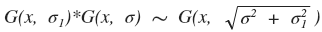and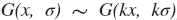Tilde means equality up to an intensity scaling constant. The constant doesn't matter in our case because we always scale the LSF to fit in range [0..1].

Using the mentioned properties we can rewrite Eq. 1: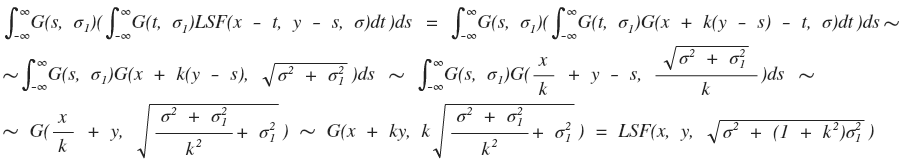Thus, applying 2d Gaussian blur with sigma =to 'ideal' slanted edge is similar to applying 1d Gaussian blur with sigma =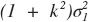to every scan line, where k is the edge slope.

Oleg Kurtsev (okurtsev@quickmtf.com)